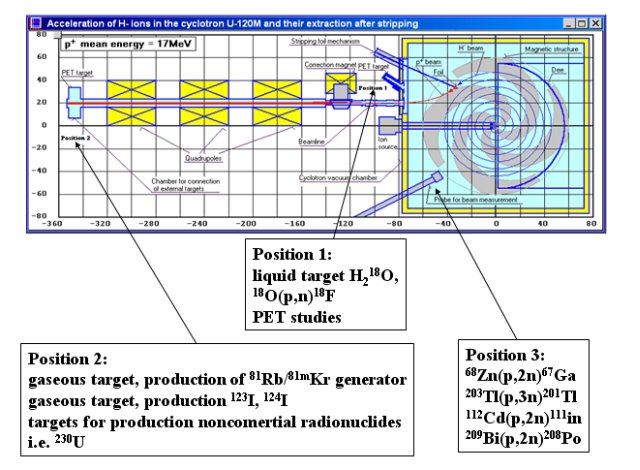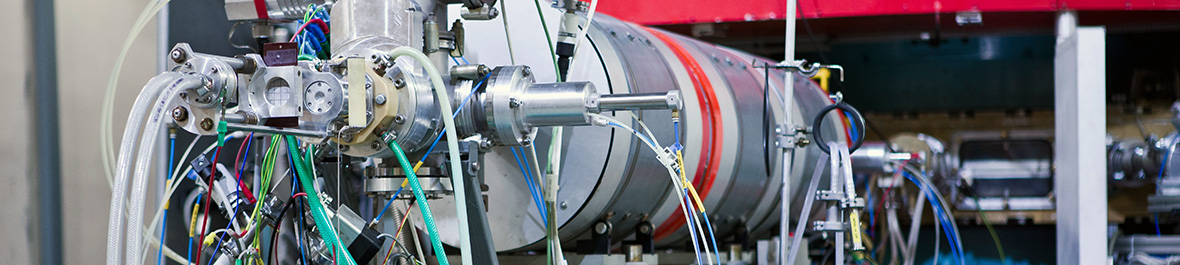# Matematický model

Mathematical simulation and analysis of the cyclotron U-120M

### Main modelling systems:

#### Matrix model of cyclotron

• computation and optimization of cyclotron parameters for arbitrary selected accelerating regime set-up via the computer control system

• numerical and graphical representation of calculated cyclotron parameters and main beam properties

• saving the data files for dynamic simulation of any accelerating regime.

#### Dynamic simulation of acceleration and extraction process

• equilibrium orbit analyses

• computation of ion trajectories in 3D space ( injection, acceleration and extraction )

• numerical and graphical representation of calculated beam properties

### Auxiliary systems:

• 2-D preliminary magnetic field topography measurement and processing

• computation of 3-D electric field of the actual accelerating structure

• interpolation of magnetic field topography for any accelerating regimeMatrix model of cyclotron
 Main goal: calculations of currents in the main and trim magnetic coils for any accelerated regime. Acquirement of a radial dependence of an average magnetic field as near as possible to the isochronous one. Optimization of beam phase radial dependencies as well as of the radial and azimutal free oscillations and the trim coils power consumption. The magnet currents are optimized by an automatically working algorithm. It is possible to minimize either the magnetic field deviations from isochronism or the beam phase history. A fine manual tuning with respect to the beam phase history and the frequency of radial and axial free oscillations is also possible. Graphical and numerical representation of acquired data. Saving the files with description of the corresponding magnetic field for an use in dynamic simulation programs.

Simplified scheme of matrix model of cyclotron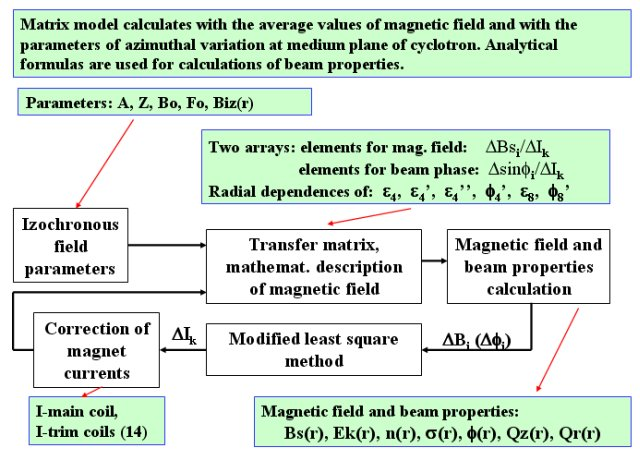Input and otput windows of matrix model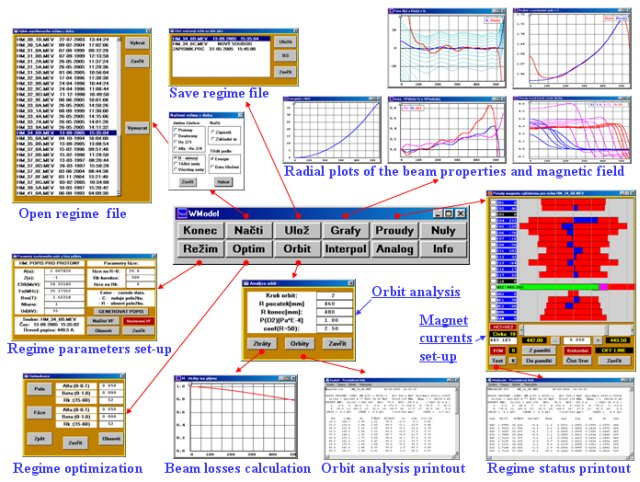Processing of Cyclotron magnetic fieldElectrostatic fieldEquilibrium orbits analysesIon beam dynamics computationSimplified scheme of beam dynamics mathematic simulationSetting up the accelerated beam parameters and program modificationNotes:
 Ions injected from an ion source are distributed regularly at the slit 1.6 x 4mm2 The contribution of each injected ion to total “beam current” depends on its starting position and the value of Dee voltage at the moment of injection (i.e. on the value of RF phase at that moment) It is also possible to inject ions at any radius with parameters obtained for example from equilibrium orbits All components of magnetic and electric field are calculated at each integration step at all region of acceleration. The loss of ion is then indicated by zero or maximal potential at actual position, corresponding either to the high voltage or to the grounded electrode The coordinate axes or the beam probes can be chosen as the beam stop lines. It is also possible to prepare the conditions for an automatic beam extraction to required point or for a fine manual tuning of extracted beam

Accelerated beam properties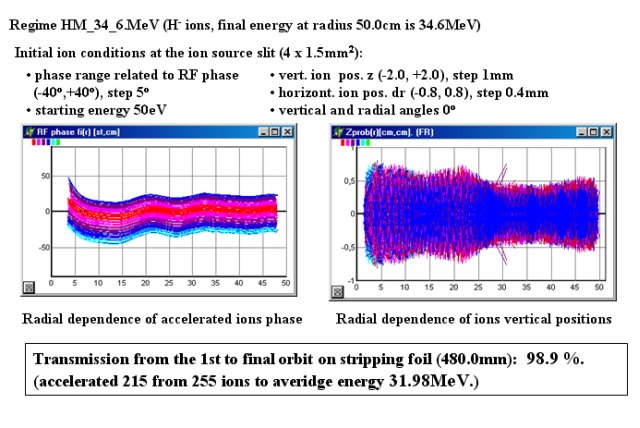Centering of accelerated beam by harmonic coilsInfluence of centering on the beam (Rstop = 48.00 cm)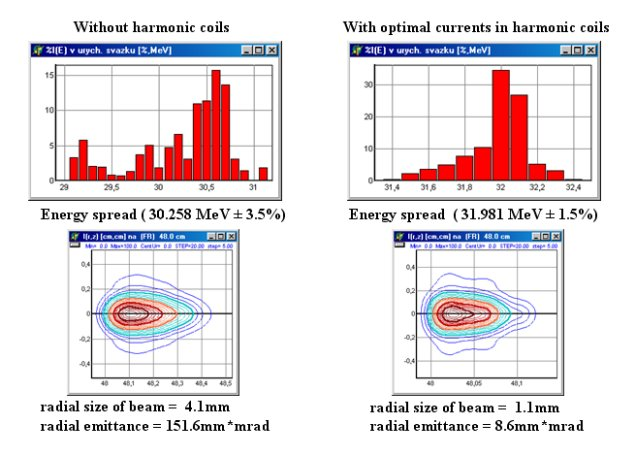Numerical output of accelerated beam properties
 *** ACCELERATED BEAM: ***Accelerated 215 from 255 ions in regime Hm_34_6b.bhp :Phase from -40o to 40o with step 5.0oZet from -2.0 to +2.0 with step 1.00mmdrIZ from -0.80 to 0.80 with step 0.40mmStarting energy of ions 0.000050 MeVNo dFi0Rstop= 48.20 cm, fiStop= 134.00oRorb= 48.34 cm, Xc= 0.19 cm, Yc= -0.09 cm.Current at 1. orbit= 1712.4, at RStop= 1693.0.Beam transfer from first orbit to RMax is 98.9 %.Average values of accelerated ions properties : Rmean= 48.24cm, radial. angle= 2.01o.Zmean= 0.00 cm, axial. angle= 0.00o.Emean= 32.240 MeV.

Analyses of the accelerated ions energy distributionAccelerated beam emittance at the stripping foil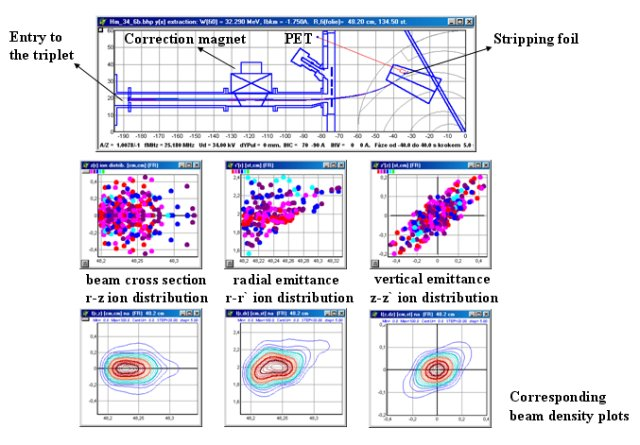Stripping method of H-/D- extractionSetting up the extraction system parametersThe magnetic field of the correction magnetExtraction of the 32.25MeV proton beam to the ion lineNumerical output of extracted beam propertiesDistribution of ions extracted to the triplet entryExtracted beam energy: calculation & foil activation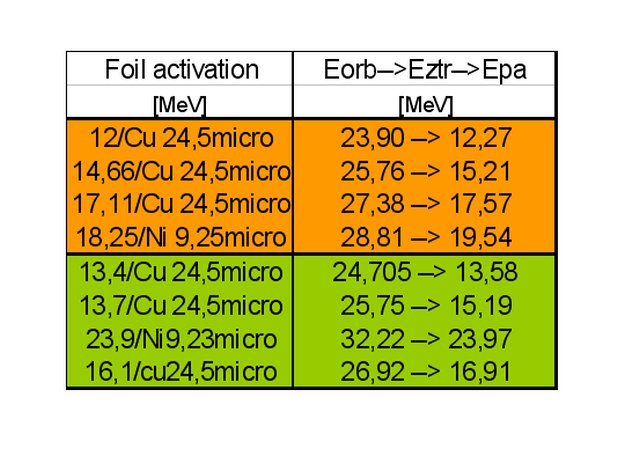Extracted beam emittance at the triplet entry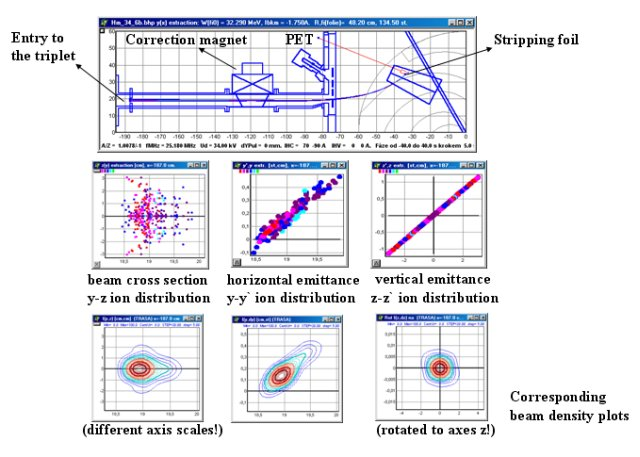Acceleration and extraction of H- and D- ions at cyclotron U-120M